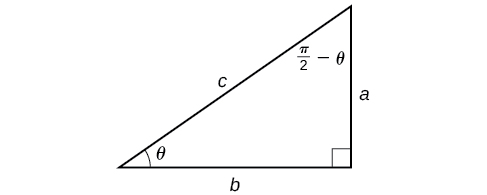# 8.3 Inverse trigonometric functions  (Page 4/15)

 Page 4 / 15

## Evaluating compositions of the form f ( f−1 ( y )) and f−1 ( f ( x ))

For any trigonometric function, $\text{\hspace{0.17em}}f\left({f}^{-1}\left(y\right)\right)=y\text{\hspace{0.17em}}$ for all $\text{\hspace{0.17em}}y\text{\hspace{0.17em}}$ in the proper domain for the given function. This follows from the definition of the inverse and from the fact that the range of $\text{\hspace{0.17em}}f\text{\hspace{0.17em}}$ was defined to be identical to the domain of $\text{\hspace{0.17em}}{f}^{-1}.\text{\hspace{0.17em}}$ However, we have to be a little more careful with expressions of the form $\text{\hspace{0.17em}}{f}^{-1}\left(f\left(x\right)\right).$

## Compositions of a trigonometric function and its inverse

$\begin{array}{l}\text{\hspace{0.17em}}\text{\hspace{0.17em}}\mathrm{sin}\left({\mathrm{sin}}^{-1}x\right)=x\text{\hspace{0.17em}}\text{for}\text{\hspace{0.17em}}-1\le x\le 1\hfill \\ \mathrm{cos}\left({\mathrm{cos}}^{-1}x\right)=x\text{\hspace{0.17em}}\text{for}\text{\hspace{0.17em}}-1\le x\le 1\hfill \\ \text{\hspace{0.17em}}\mathrm{tan}\left({\mathrm{tan}}^{-1}x\right)=x\text{\hspace{0.17em}}\text{for}\text{\hspace{0.17em}}-\infty

Is it correct that $\text{\hspace{0.17em}}{\mathrm{sin}}^{-1}\left(\mathrm{sin}\text{\hspace{0.17em}}x\right)=x?$

No. This equation is correct if $\text{\hspace{0.17em}}x\text{\hspace{0.17em}}$ belongs to the restricted domain $\text{\hspace{0.17em}}\left[-\frac{\pi }{2},\frac{\pi }{2}\right],\text{\hspace{0.17em}}$ but sine is defined for all real input values, and for $\text{\hspace{0.17em}}x\text{\hspace{0.17em}}$ outside the restricted interval, the equation is not correct because its inverse always returns a value in $\text{\hspace{0.17em}}\left[-\frac{\pi }{2},\frac{\pi }{2}\right].\text{\hspace{0.17em}}$ The situation is similar for cosine and tangent and their inverses. For example, $\text{\hspace{0.17em}}{\mathrm{sin}}^{-1}\left(\mathrm{sin}\left(\frac{3\pi }{4}\right)\right)=\frac{\pi }{4}.$

Given an expression of the form f −1 (f(θ)) where evaluate.

1. If $\text{\hspace{0.17em}}\theta \text{\hspace{0.17em}}$ is in the restricted domain of
2. If not, then find an angle $\text{\hspace{0.17em}}\varphi \text{\hspace{0.17em}}$ within the restricted domain of $\text{\hspace{0.17em}}f\text{\hspace{0.17em}}$ such that $\text{\hspace{0.17em}}f\left(\varphi \right)=f\left(\theta \right).\text{\hspace{0.17em}}$ Then $\text{\hspace{0.17em}}{f}^{-1}\left(f\left(\theta \right)\right)=\varphi .$

## Using inverse trigonometric functions

Evaluate the following:

1. ${\mathrm{sin}}^{-1}\left(\mathrm{sin}\left(\frac{\pi }{3}\right)\right)$
2. ${\mathrm{sin}}^{-1}\left(\mathrm{sin}\left(\frac{2\pi }{3}\right)\right)$
3. ${\mathrm{cos}}^{-1}\left(\mathrm{cos}\left(\frac{2\pi }{3}\right)\right)$
4. ${\mathrm{cos}}^{-1}\left(\mathrm{cos}\left(-\frac{\pi }{3}\right)\right)$
1. so $\text{\hspace{0.17em}}{\mathrm{sin}}^{-1}\left(\mathrm{sin}\left(\frac{\pi }{3}\right)\right)=\frac{\pi }{3}.$
2. but $\text{\hspace{0.17em}}\mathrm{sin}\left(\frac{2\pi }{3}\right)=\mathrm{sin}\left(\frac{\pi }{3}\right),\text{\hspace{0.17em}}$ so $\text{\hspace{0.17em}}{\mathrm{sin}}^{-1}\left(\mathrm{sin}\left(\frac{2\pi }{3}\right)\right)=\frac{\pi }{3}.$
3. so $\text{\hspace{0.17em}}{\mathrm{cos}}^{-1}\left(\mathrm{cos}\left(\frac{2\pi }{3}\right)\right)=\frac{2\pi }{3}.$
4. but $\text{\hspace{0.17em}}\mathrm{cos}\left(-\frac{\pi }{3}\right)=\mathrm{cos}\left(\frac{\pi }{3}\right)\text{\hspace{0.17em}}$ because cosine is an even function.
5. so $\text{\hspace{0.17em}}{\mathrm{cos}}^{-1}\left(\mathrm{cos}\left(-\frac{\pi }{3}\right)\right)=\frac{\pi }{3}.$

Evaluate $\text{\hspace{0.17em}}{\mathrm{tan}}^{-1}\left(\mathrm{tan}\left(\frac{\pi }{8}\right)\right)\text{\hspace{0.17em}}\text{and}\text{\hspace{0.17em}}{\mathrm{tan}}^{-1}\left(\mathrm{tan}\left(\frac{11\pi }{9}\right)\right).$

$\frac{\pi }{8};\frac{2\pi }{9}$

## Evaluating compositions of the form f−1 ( g ( x ))

Now that we can compose a trigonometric function with its inverse, we can explore how to evaluate a composition of a trigonometric function and the inverse of another trigonometric function. We will begin with compositions of the form $\text{\hspace{0.17em}}{f}^{-1}\left(g\left(x\right)\right).\text{\hspace{0.17em}}$ For special values of $\text{\hspace{0.17em}}x,$ we can exactly evaluate the inner function and then the outer, inverse function. However, we can find a more general approach by considering the relation between the two acute angles of a right triangle where one is $\text{\hspace{0.17em}}\theta ,\text{\hspace{0.17em}}$ making the other $\text{\hspace{0.17em}}\frac{\pi }{2}-\theta .$ Consider the sine and cosine of each angle of the right triangle in [link] .Right triangle illustrating the cofunction relationships

Because $\text{\hspace{0.17em}}\mathrm{cos}\text{\hspace{0.17em}}\theta =\frac{b}{c}=\mathrm{sin}\left(\frac{\pi }{2}-\theta \right),\text{\hspace{0.17em}}$ we have $\text{\hspace{0.17em}}{\mathrm{sin}}^{-1}\left(\mathrm{cos}\text{\hspace{0.17em}}\theta \right)=\frac{\pi }{2}-\theta \text{\hspace{0.17em}}$ if $\text{\hspace{0.17em}}0\le \theta \le \pi .\text{\hspace{0.17em}}$ If $\text{\hspace{0.17em}}\theta \text{\hspace{0.17em}}$ is not in this domain, then we need to find another angle that has the same cosine as $\text{\hspace{0.17em}}\theta \text{\hspace{0.17em}}$ and does belong to the restricted domain; we then subtract this angle from $\text{\hspace{0.17em}}\frac{\pi }{2}.$ Similarly, $\text{\hspace{0.17em}}\mathrm{sin}\text{\hspace{0.17em}}\theta =\frac{a}{c}=\mathrm{cos}\left(\frac{\pi }{2}-\theta \right),\text{\hspace{0.17em}}$ so $\text{\hspace{0.17em}}{\mathrm{cos}}^{-1}\left(\mathrm{sin}\text{\hspace{0.17em}}\theta \right)=\frac{\pi }{2}-\theta \text{\hspace{0.17em}}$ if $\text{\hspace{0.17em}}-\frac{\pi }{2}\le \theta \le \frac{\pi }{2}.\text{\hspace{0.17em}}$ These are just the function-cofunction relationships presented in another way.

Given functions of the form $\text{\hspace{0.17em}}{\mathrm{sin}}^{-1}\left(\mathrm{cos}\text{\hspace{0.17em}}x\right)\text{\hspace{0.17em}}$ and $\text{\hspace{0.17em}}{\mathrm{cos}}^{-1}\left(\mathrm{sin}\text{\hspace{0.17em}}x\right),\text{\hspace{0.17em}}$ evaluate them.

1. If then $\text{\hspace{0.17em}}{\mathrm{sin}}^{-1}\left(\mathrm{cos}\text{\hspace{0.17em}}x\right)=\frac{\pi }{2}-x.$
2. If then find another angle such that $\text{\hspace{0.17em}}\mathrm{cos}\text{\hspace{0.17em}}y=\mathrm{cos}\text{\hspace{0.17em}}x.$
${\mathrm{sin}}^{-1}\left(\mathrm{cos}\text{\hspace{0.17em}}x\right)=\frac{\pi }{2}-y$
3. If then $\text{\hspace{0.17em}}{\mathrm{cos}}^{-1}\left(\mathrm{sin}\text{\hspace{0.17em}}x\right)=\frac{\pi }{2}-x.$
4. If then find another angle such that $\text{\hspace{0.17em}}\mathrm{sin}\text{\hspace{0.17em}}y=\mathrm{sin}\text{\hspace{0.17em}}x.$
${\mathrm{cos}}^{-1}\left(\mathrm{sin}\text{\hspace{0.17em}}x\right)=\frac{\pi }{2}-y$

#### Questions & Answers

write down the polynomial function with root 1/3,2,-3 with solution
if A and B are subspaces of V prove that (A+B)/B=A/(A-B)
write down the value of each of the following in surd form a)cos(-65°) b)sin(-180°)c)tan(225°)d)tan(135°)
Prove that (sinA/1-cosA - 1-cosA/sinA) (cosA/1-sinA - 1-sinA/cosA) = 4
what is the answer to dividing negative index
In a triangle ABC prove that. (b+c)cosA+(c+a)cosB+(a+b)cisC=a+b+c.
give me the waec 2019 questions
the polar co-ordinate of the point (-1, -1)
prove the identites sin x ( 1+ tan x )+ cos x ( 1+ cot x )= sec x + cosec x
tanh`(x-iy) =A+iB, find A and B
B=Ai-itan(hx-hiy)
Rukmini
what is the addition of 101011 with 101010
If those numbers are binary, it's 1010101. If they are base 10, it's 202021.
Jack
extra power 4 minus 5 x cube + 7 x square minus 5 x + 1 equal to zero
the gradient function of a curve is 2x+4 and the curve passes through point (1,4) find the equation of the curve
1+cos²A/cos²A=2cosec²A-1
test for convergence the series 1+x/2+2!/9x3ByByByBy Dravida Mahadeo-J...By